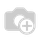• Cenník

# How to find out number of hours between two datetime object?

'date_from':fields.datetime('Date From'),
'date_to':fields.datetime('Date To'),

d_frm_obj = datetime.datetime.strptime(date_from, DEFAULT_SERVER_DATETIME_FORMAT)
d_to_obj = datetime.datetime.strptime(date_to, DEFAULT_SERVER_DATETIME_FORMAT)
temp_date = d_to_obj-d_frm_obj.hours         (This got errors)

From the above code  How to find out the number of hours between these two objects...need a help

2 KomentáreHi, Just write down as like below. *temp_date = d_to_obj-d_frm_obj* *diff_in_sec = temp_date.total_seconds()* *diff_in_hours = diff_in_sec / 60* I hope you will get the difference.

##### 2 Odpovede

Try this

d_frm_obj = datetime.strptime(date_from, DEFAULT_SERVER_DATETIME_FORMAT)
d_to_obj = datetime.strptime(date_to, DEFAULT_SERVER_DATETIME_FORMAT)
temp_date = d_to_obj - d_frm_obj
print temp_date.seconds/3600       //This will provide hours.
print (diff.days *24) + (diff.seconds/3600)    //Provides total number of hours including days

For more info: http://stackoverflow.com/a/2119512/2470366

1 KomentárHello Libu

d_frm_obj = datetime.datetime.strptime(date_from, DEFAULT_SERVER_DATETIME_FORMAT)
d_to_obj = datetime.datetime.strptime(date_to, DEFAULT_SERVER_DATETIME_FORMAT)

diff = d_to_obj - d_frm_obj

hours = (diff.seconds)/ 3600
print hours

diff_days = diff.days
print diff_days

days_hours = diff_days * 24
print days_hours

total_hours = days_hours + hours
print total_hours

Hope this helps !!